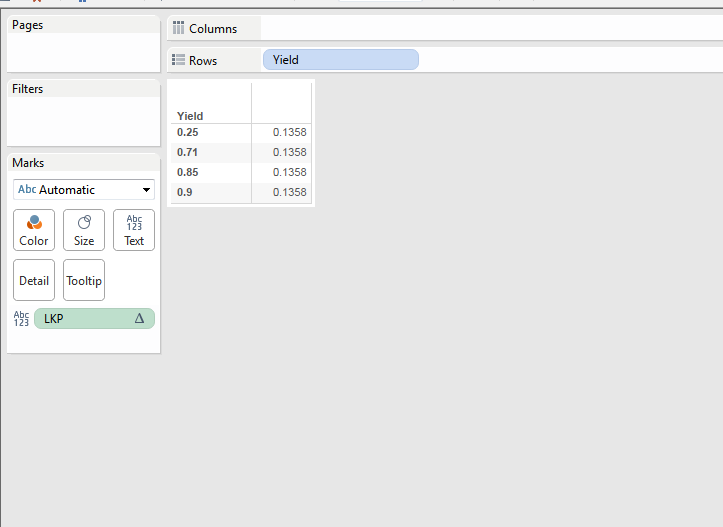# How to do a product of all rows in a column in Tableau

1 view
in BI

I have a column as below. I want to multiply all values together and get one value across

Yield

0.90

0.85

0.71

0.25

Yield Total_Prod

0.90   0.135

0.85   0.135

0.71   0.135

0.25   0.135

Where Total_Prod = 0.90*0.85*0.71*0.25

by (17.6k points)
• Running product is given by this calculation. Refer to this Link

[RP] : EXP(RUNNING_SUM(LN(SUM([Yield]))))

• But In order to find the Total Product use this calculation :

LOOKUP([RP],LAST())

To get the output like this: